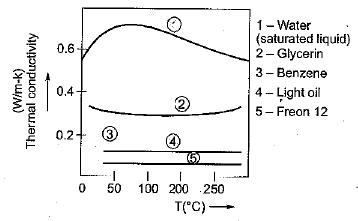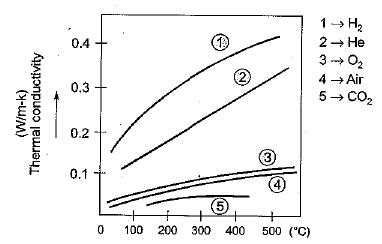Courses

# Test: Basic Concepts of Heat Transfer - 2

## 10 Questions MCQ Test Topicwise Question Bank for Mechanical Engineering | Test: Basic Concepts of Heat Transfer - 2

Description
This mock test of Test: Basic Concepts of Heat Transfer - 2 for Chemical Engineering helps you for every Chemical Engineering entrance exam. This contains 10 Multiple Choice Questions for Chemical Engineering Test: Basic Concepts of Heat Transfer - 2 (mcq) to study with solutions a complete question bank. The solved questions answers in this Test: Basic Concepts of Heat Transfer - 2 quiz give you a good mix of easy questions and tough questions. Chemical Engineering students definitely take this Test: Basic Concepts of Heat Transfer - 2 exercise for a better result in the exam. You can find other Test: Basic Concepts of Heat Transfer - 2 extra questions, long questions & short questions for Chemical Engineering on EduRev as well by searching above.
QUESTION: 1

### Thermal conductivity of water in general with rise in temperature

Solution:QUESTION: 2

### Thermal conductivity of air with rise in temperature

Solution:QUESTION: 3

### Heat is mainly transferred by conduction, convection and radiation in

Solution:

Because for radiation to be comparable the magnitude of temperature difference should be large enough, Convection & conduction is also predominant in boiler furnace.

QUESTION: 4

Heat conduction in gases is due to

Solution:
QUESTION: 5

Heat transfer by molecular collision in

Solution:
QUESTION: 6

Arrangement of silver, air, aluminium and lead in order of increasing thermal conductivity at room temperature yields

Solution:

According to their thermal conductivity for Air, k = 0.022 W/mK
for Aluminium, k = 205 W/mK
for Silver, k = 407 W/mK
Hence correct sequence is
Air, Lead, Aluminium, Silve

QUESTION: 7

Metals are good conductors of heat because of

Solution:
QUESTION: 8

Choose the correct statement about thermaf conductivity

Solution:

Due to increase in lattice vibratioh the thermal conductivity of metals decreases with increase in temperature.

QUESTION: 9

Cork is used as a good insulator

Solution:
QUESTION: 10

In which one of the following materials, is the heat energy propagation minimum due to conduction heat transfer

Solution:

Since thermal conductivity of air is minimum among the given material hence heat transfer will be minimum in air.